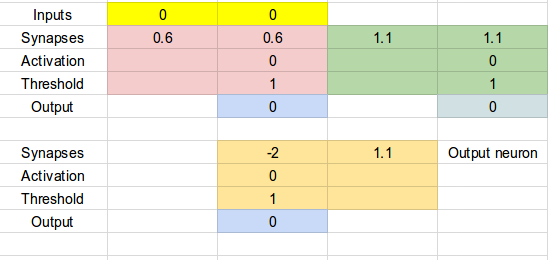Original article was published by Moral Robots on Artificial Intelligence on Medium## Your own neural network on the cheap

In the previous post, we saw what a neural network is and how it works. Now comes the fun part. We’ll make one in a spreadsheet.

It doesn’t matter which spreadsheet you use. You can visualise such neurons in Excel, or you can equally well use LibreOffice, Google Sheets or any other spreadsheet application.

In the top line, the two yellow cells are the input values. You can change these to simulate various inputs. The next line contains the two synaptic weights by which we will multiply the input values. Here we put them to 0.6 to simulate a logical and. Like above, if you change both synaptic weights to 1.1, you will make the neuron behave like a logical or, since the threshold is put at 1, and if either input is 1.1, and thus greater than the threshold value, the neuron will fire. In the next line, we see the activation value, which is just the sum of the two weighted inputs. The next line contains the threshold, which in all these examples is 1. But there is no reason not to change it to any other value if you want to experiment. Finally, in the last line, the blue cell contains the output of the neuron. This will be 1 if the neuron has fired or 0 if the neuron has not fired.

You can also easily add additional neurons to your network and try to create more complex behaviours.

The only special thing you need to do is to insert two formulas into the spreadsheet:

1. Into cell C3 (the “activation” cell), we enter the formula for calculating the weighted sum of the two inputs: =(D1*D2)+(B1*B2).
2. Into cell C5 (“output”), we enter a conditional statement: =if(C3>=C4, 1, 0). This is saying: if the sum of the inputs (cell C3) is greater than the threshold (cell C4), the neuron will fire (output=1); otherwise, it will stay silent (output=0). These formulas are written for Google Sheets, so if you use another spreadsheet application, you might need to change the syntax a bit.

That’s it! Your first, very own artificial neuron. You can now play with it. You can change the synaptic weights and the activation thresholds, and observe how it works. You can also easily add additional neurons to your network and try to create more complex behaviours. Of course, this doesn’t yet learn. It is pre-programmed by the fixed synaptic weights to do one thing only.

As an additional example, let’s look at how we would create a little network that can calculate the ‘exclusive or’, or xor, function in a spreadsheet. Here is the network we want to simulate (we discussed how this works in the previous post):

And here is the same neural network in a spreadsheet table. Again, the input is on top (yellow), and the output is at the bottom (blue). But now we have three neurons. The first layer (above) consists of two neurons (pink and green). Each of these neurons is connected to the two yellow inputs; this is why each has two synapses instead of one. Each of these neurons has its own (blue) output. Both intermediate outputs from the first layer feed into the orange neuron at the bottom. The orange neuron’s inputs are the outputs of the previous layer; this is why we don’t have yellow input cells for that neuron. The output of the whole network is the blue cell at the very bottom.

This neuron will implement an xor function between its (yellow) inputs in the first row and the blue output cell at the very bottom:

What you can see from this, is how the behaviour of a neural network is all encoded in the synaptic weights, which, in this case, we entered by hand. In a later post will see how an artificial neural network can change these weights by itself, and in this way learn new behaviours. Make sure to follow this series, so you don’t miss the fun!

Simulating neural networks in a spreadsheet is a great way to learn how they work and to get accustomed to the basic ideas and the structure of artificial neurons. I hope you enjoyed this and I hope to see you around here next time!